Math Olympiad Test: Co-ordinate Geometry- 2

# Math Olympiad Test: Co-ordinate Geometry- 2

Test Description

## 15 Questions MCQ Test Mathematics Olympiad for Class 9 | Math Olympiad Test: Co-ordinate Geometry- 2

Math Olympiad Test: Co-ordinate Geometry- 2 for Class 9 2022 is part of Mathematics Olympiad for Class 9 preparation. The Math Olympiad Test: Co-ordinate Geometry- 2 questions and answers have been prepared according to the Class 9 exam syllabus.The Math Olympiad Test: Co-ordinate Geometry- 2 MCQs are made for Class 9 2022 Exam. Find important definitions, questions, notes, meanings, examples, exercises, MCQs and online tests for Math Olympiad Test: Co-ordinate Geometry- 2 below.
Solutions of Math Olympiad Test: Co-ordinate Geometry- 2 questions in English are available as part of our Mathematics Olympiad for Class 9 for Class 9 & Math Olympiad Test: Co-ordinate Geometry- 2 solutions in Hindi for Mathematics Olympiad for Class 9 course. Download more important topics, notes, lectures and mock test series for Class 9 Exam by signing up for free. Attempt Math Olympiad Test: Co-ordinate Geometry- 2 | 15 questions in 15 minutes | Mock test for Class 9 preparation | Free important questions MCQ to study Mathematics Olympiad for Class 9 for Class 9 Exam | Download free PDF with solutions
 1 Crore+ students have signed up on EduRev. Have you?
Math Olympiad Test: Co-ordinate Geometry- 2 - Question 1

### The point of intersection of both the axes is called:

Detailed Solution for Math Olympiad Test: Co-ordinate Geometry- 2 - Question 1

The horizontal axis in the coordinate plane is called the x-axis. The vertical axis is called the y-axis. The point at which the two axes intersect is called the origin.

Math Olympiad Test: Co-ordinate Geometry- 2 - Question 2

### If two points P and Q have same abscissae and different ordinates, then points P and Q will definitely lie on

Detailed Solution for Math Olympiad Test: Co-ordinate Geometry- 2 - Question 2

If P and Q have same abscissa but different ordinates then P and Q have coordinates, as P (a, c ), Q (a, b)
∵ x-coordinate is constant.
∴ P and Q will lie on line parallel to y-axis.

Math Olympiad Test: Co-ordinate Geometry- 2 - Question 3

### Minimum distance of point (4, 6) from x -axis will be

Detailed Solution for Math Olympiad Test: Co-ordinate Geometry- 2 - Question 3

Minimum distance of point (α, b) from x-axis = Perpendicular distance between point and x-axis = |y-coordinate (ordinate) of the point| = |6| = 6

Math Olympiad Test: Co-ordinate Geometry- 2 - Question 4

Point P (4, -3) will lie in:

Detailed Solution for Math Olympiad Test: Co-ordinate Geometry- 2 - Question 4

Point (4, 3) have (+, -) sign convention, that belongs to IVth quadrant.

Math Olympiad Test: Co-ordinate Geometry- 2 - Question 5

If two points M and N lie on y-axis, and have same absolute value of abscissa but different signs. If the abscissa of point M is K, then the distance between M and N is equal to:

Detailed Solution for Math Olympiad Test: Co-ordinate Geometry- 2 - Question 5

Let the coordinates of point M be (0, k)
{∵ M is on y-axis}
∴ Coordinates of point N = (0, - K)
∴ Distance between point M and N
= |K - (-K)| = 2|K|

Math Olympiad Test: Co-ordinate Geometry- 2 - Question 6

Point (-3, -2) will lie in:

Detailed Solution for Math Olympiad Test: Co-ordinate Geometry- 2 - Question 6

∵ (-3, -2) has (-, -) sign convention.
∴ (-3, -2)/0 Belongs to 3rd quadrant.

Math Olympiad Test: Co-ordinate Geometry- 2 - Question 7

The mirror image of point (-4, -2) about x-axis will lie in:

Detailed Solution for Math Olympiad Test: Co-ordinate Geometry- 2 - Question 7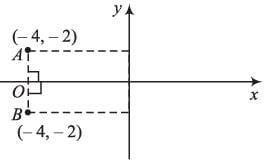Point A is the reflected point.
Point A will have coordinates, as, abscissa will not change and ordinate will change sign
∴ Reflected point will lie in 2nd quadrant.

Math Olympiad Test: Co-ordinate Geometry- 2 - Question 8

The distance between (12, 5) and origin is

Detailed Solution for Math Olympiad Test: Co-ordinate Geometry- 2 - Question 8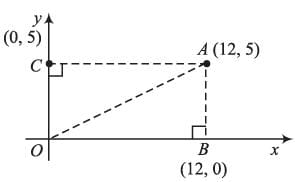In ΔOAB
OA2 = OB2 + AB2
= (12)2 + (OC)2 = (12)2 + (5)2
= 169
⇒ OA = √169 = 13 Units

Math Olympiad Test: Co-ordinate Geometry- 2 - Question 9

Equation of y-axis will be

Detailed Solution for Math Olympiad Test: Co-ordinate Geometry- 2 - Question 9

On every point of y-axis, abscissa = 0
∴ x =  0 as the equation of y-axis

Math Olympiad Test: Co-ordinate Geometry- 2 - Question 10

The area of triangle formed by points A (3, 0), B (0, -4), C (0, 4) will be (in sq. units)

Detailed Solution for Math Olympiad Test: Co-ordinate Geometry- 2 - Question 10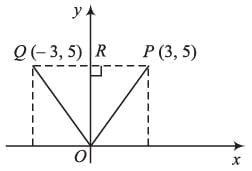Coordinates of point Q ≡ (-3, 5).
After plotting ΔPOQ on graph, it can be clearly viewed that ΔPOQ is isosceles.
∴ Area of ΔPOQ = 1/2 × OR × PQ
= 1/2 × 5 × [3- (-3)]
= 1/2 × 5 × 6 = 15 sq. units

Math Olympiad Test: Co-ordinate Geometry- 2 - Question 11

Distance between points (24, 10) and (-48, 10) will be

Detailed Solution for Math Olympiad Test: Co-ordinate Geometry- 2 - Question 11

Distance between (-24, 10) and (48,10) will be equal to the absolute value of difference between the abscissa of the points as, the points have same ordinate.
∴ Distance = |48 - (-24)| = 72 units

Math Olympiad Test: Co-ordinate Geometry- 2 - Question 12

The difference between ordinates of point P (3, -6) and Q (-6, 3) is

Detailed Solution for Math Olympiad Test: Co-ordinate Geometry- 2 - Question 12

Ordinates of points are -6 and 3
∴ Difference = -6 - 3 = -9

Math Olympiad Test: Co-ordinate Geometry- 2 - Question 13

The point of intersection of lines having equations x + y = 6 and x - y = 2, is

Detailed Solution for Math Olympiad Test: Co-ordinate Geometry- 2 - Question 13

Let the coordinates of point of intersection be (x1, y1)
∴ x1 + y1 = 6
x1 - y1 = 2
Solving these two equations, we get
x1 = 4, and , y1 = 2
∴ point of intersection  ≡ (4, 2)

Math Olympiad Test: Co-ordinate Geometry- 2 - Question 14

Distance of point (- 24 ,10) from origin will be

Detailed Solution for Math Olympiad Test: Co-ordinate Geometry- 2 - Question 14

In ΔOBC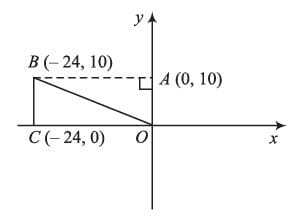BC2 + OC2 = OB2
⇒ (OA)2 + (OC)2 = OB2
⇒ (10)2 + (24)2 = OB2
⇒ OB = √676 = 26 units

Math Olympiad Test: Co-ordinate Geometry- 2 - Question 15

The area of triangle formed by the points A (2, 0), B (6, 0), C (4, 6) is

Detailed Solution for Math Olympiad Test: Co-ordinate Geometry- 2 - Question 15

After plotting figure, it can be clearly seen that ABC is an isosceles triangle in which,
AB = (6 - 2) = 4 units
CD = (6 - 0) = 6 units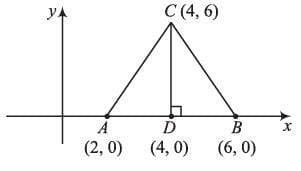∴ Ares of ΔABC = 1/2 × AB × CD
= 1/2 × 4 × 6 = 12 sq. units

## Mathematics Olympiad for Class 9

1 videos|43 tests
 Use Code STAYHOME200 and get INR 200 additional OFF Use Coupon Code
Information about Math Olympiad Test: Co-ordinate Geometry- 2 Page
In this test you can find the Exam questions for Math Olympiad Test: Co-ordinate Geometry- 2 solved & explained in the simplest way possible. Besides giving Questions and answers for Math Olympiad Test: Co-ordinate Geometry- 2, EduRev gives you an ample number of Online tests for practice

## Mathematics Olympiad for Class 9

1 videos|43 tests